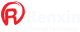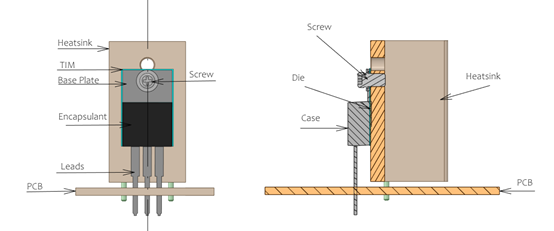#Misunderstandings about the selection of the Heatsink, Electronic engineers easily overlooked details
How to Select a Heat Sink
How to Select a Heat Sink?

Heatsink` are an important element in circuit design because they provide an efficient path for heat to be transferred into the ambient air and away from electronic devices (eg. BJTs, MOSFETs, linear regulators, etc.). The general  philosophy behind a heat sink is to increase the surface area of the heat-producing device, enabling a more efficient transfer of heat into the ambient environment. This improved thermal pathway reduces the temperature rise in the junction of the electronic device. The following post is intended to provide high-level primer on heat sink selection utilizing thermal data from your application and specifications from the heat sink vendor.

Is a Heat Sink Required?
For the remainder of this post, let us assume that an application is being developed using a transistor housed in a TO-220 package, the switching and conduction losses of the transistor equate to a power dissipation of 2.78 W, and the ambient operating temperature of this application is not expected to exceed 50°C. Will a heat sink be required for this transistor?Front and side view drawings of a typical TO-220 package with heat sink

The first step is to gather and understand all of the thermal impedances that stand in the way of the 2.78 W from being dissipated into the ambient air. If those Watts cannot dissipate efficiently, the junction temperature inside the TO-220 package will rise beyond the recommended operating conditions (typically 125°C for silicon). Most transistor vendors will document a “junction-to-ambient” thermal impedance denoted by the symbol Rθ J-A, which is measured in units of °C/W. This value represents how much the junction temperature will rise above the ambient temperature surrounding the TO-220 package for every Watt of power dissipated within the device.

For example, if the transistor vendor states that the thermal impedance from junction-to-ambient is 62°C/W, this means that the 2.78 W being dissipated within the TO-220 package will cause the junction temperature to rise 172°C above ambient (calculated as 2.78 W x 62°C/W). Assuming the worst-case ambient temperature for this application of 50°C, the junction temperature will reach 222°C (calculated as 50°C + 172°C). This far exceeds the rated silicon temperature of 125°C and will permanently damage the transistor. Hence, a heat sink is required. Attaching a heat sink will lower the junction-to-ambient thermal impedance significantly. The next step will be to determine how low the thermal impedance path needs to be for safe and reliable operation.

Determining Thermal Impedance Paths
To do this, start with the largest permissible temperature rise. If the maximum ambient operating temperature of the application is 50°C and the silicon junction must remain at 125°C or less, the largest permissible temperature rise is 75°C (calculated as 125°C - 50°C).
Next, calculate the largest permissible thermal impedance between the junction and ambient air. If the largest permissible temperature rise is 75°C and the Watts dissipated in the TO-220 package is 2.78 W, the largest permissible thermal impedance would be 27°C/W (calculated as 75°C ÷ 2.78 W).
Finally, add up all the thermal impedance paths from the silicon junction to the ambient air and ensure they sum up to less than the largest permissible thermal impedance; 27°C/W in this example